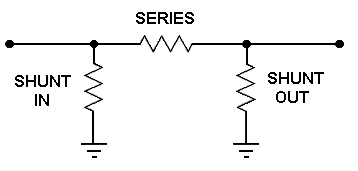# Pi Attenuator Calculator

Calculates the resistor values, attenuation, 'impedance', reflection coefficient, VSWR and return loss of a Pi attenuator. This can be built into a FLEXI-BOX and a transmission line PCB is available which easily adapts for this circuit with one simple trimming operation. There is also a selection of suitable RF connectors available.1) Enter the system impedance and required attenuation then then press "Calculate" below to obtain the ideal resistor values. System impedance: Ohms Ideal series resistor: Ohms Required attenuation: dB Ideal shunt resistor: Ohms 2) Change the calculated resistor values in the amber text boxes below to the nearest Preferred Resistor Values or to those available then press " Calculate" below to get the resulting attenuator parameters. Preferred value of series resistor: Ohms Attenuation: dB Preferred value of shunt resistor: Ohms Input Impedance: Ohms Mismatch Losses: Included Reflection Coefficient: VSWR: Return Loss: dB

This calculator uses Visual Basic and will work in Internet Explorer and other browsers with Visual Basic plug-ins.